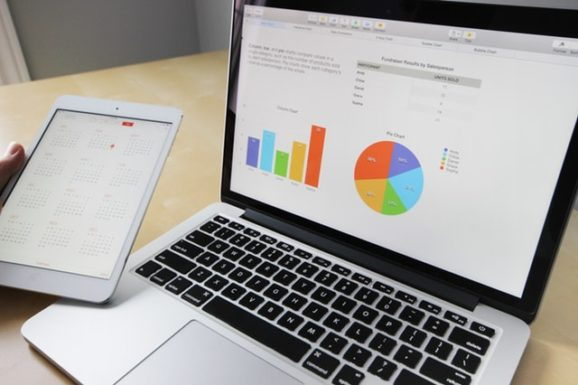Course Details

In Microsoft Excel, in order to automate many of the repetitive tasks that are part of spreadsheet development, you can simplify your work by creating macro using Excel VBA (Visual Basic for Applications) This course will cover in depth on VBA such as create user form application, design your own toolbar, shortcut menu, add-ins and help menu, apply VBA in Excel chart and pivot table for powerful data analysis result. You will be able to use the skills you learn to apply in your real life projects to fit your professional needs.

Prerequisites

Target Audience

Accountants, Professionals using Excel, Students and School Teachers, Data Analyst

Course Outline:

Lesson 1: Introduction to VBA

• Microsoft Visual Basic Fundamentals
• Microsoft Visual Basic Interface
• VBA in Visual Basic
• Macros
• Writing Codes

Lesson 2: Variables and Data Types

• Variables
• Data types
• Integral Numeric Variables
• Decimal Variables
• Any-type Variables
• The scope or lifetime of a variable
• The access level of a global variable

Lesson 3: VBA operators and operands

• VBA operators
• Arithmetic operators

Lesson 4: The Properties Window

• The appearance of properties window
• Types of properties

Lesson 5: Introduction to Forms

• Forms Fundamentals
• Using forms
• The characteristic of a form

Lesson 6: Introduction to Controls

• Controls Fundamentals
• Adding a control to a Container
• Control Selection

Lesson 7: Introduction to Conditions

• Introduction to Boolean Values
• Boolean Values and Procedures
• Boolean Built-in Functions
• Logical Operators
• Checking Whether a condition is TRUE /FALSE
• What ELSE the condition is TRUE/FALSE

Lesson 8: Functional Conditions

• Alternatives to conditions being TRUE/FALSE
• Conditional statements and functions
• If-condition Built-in Functions

Lesson 9: Conditional Selections

• The Select case statement
• Managing conditional statements
• Loop Repeater
• Loop Counters
• Exiting a procedure or a loop

Lesson 10: Introduction to built-in Functions

• Constants, expressions and formulas
• Fundamentals of Built-in Functions
• Accessory built-in functions

Lesson 11: Procedures

• Introduction to Procedures
• Procedures
• Introduction to sub-procedures
• Calling a sub-procedure
• Procedures and Access levels

Lesson 12: Introduction to Functions

• Creating a Functions
• Returning a value from a function

Lesson 13: Arguments and Parameters

• Passing arguments
• Techniques of passing arguments

Lesson 14: Strings

• Introduction to Strings
• Introduction to Characters
• Character, Strings and Procedures
• Characters and String Conversions
• The Sub-string of a String
• Other operations on Strings
• The message box
• The input box

Lesson 15: Dates and Times in VBA Excel

• Fundamentals of Dates
• The Components of a Date
• Formatting a date value
• Built-in Time Functions
• The components of a time value
• Operations on date and time values

Lesson 16: Error Handling

• Handling Errors
• In case of errors
• Types of Errors
• The Err Object

FINAL PROJECT: Creating a VBA Project with the ff. Criteria

• Excel Data Source
• Variables and Data Types
• Sequential Programming (Basic Functions)
• Selection Programming
• Iteration Programming
• User Form to Excel Transaction includes (Add, Edit, and Delete)
• Error Trapping
• Basic Report Consolidation